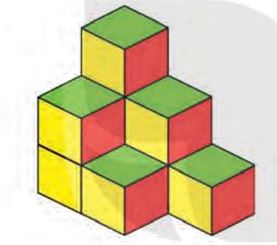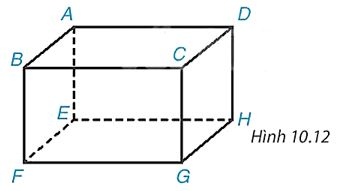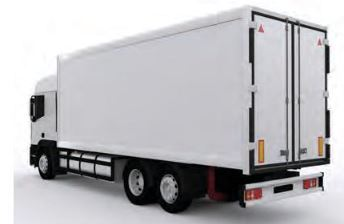## Solve Exercises Lesson 36 Rectangle and cube (Chapter 10 Math 7 Connect) – Math Book

Solve Exercises Lesson 36 Rectangles and cubes (Chapter 10 Math 7 Connect)
============

### Solution 10.1 page 90 Math 7 textbook Connecting knowledge volume 2 – KNTT

How many small cubes are there in Figure 10.11?Detailed instructions for solving problems 10.1

Solution method

Count the pictures and answer the questions

Detailed explanation

There are 9 small cubes in all.

–>

— *****

### Solve problem 10.2 page 90 Math textbook 7 Connecting knowledge volume 2 – KNTT

Name the vertices, sides, diagonals, faces of the rectangular box in Figure 10.12Detailed instructions for solving problem 10.2

Solution method

– A rectangular box has 6 faces that are rectangles, 8 vertices, 12 sides, 4 diagonals, parallel and equal sides.

– A cube is a rectangular box with 6 faces that are squares.

Detailed explanation

+ 8 vertices: A, B, C, D, E, F, G, H.

+ 12 edges: AB, AD, DC, BC, EF, EH, FG, GH, AE, BF, CG, DH

+ 4 diagonals: AG, CE, BH, DF

+ 8 sides: ABFE, DCGH, BCGF, ADHE, ABCD, EFGH.

–>

— *****

### Solution 10.4 page 91 Math 7 Textbook Connecting knowledge volume 2 – KNTT

A refrigerated truck has a rectangular box with dimensions of 5.6 m long, 2 m wide and 2 m high. Calculate the volume of the container.Detailed instructions for solving problems 10.3

Solution method

Volume of rectangular box \(V = abc\).

Detailed explanation

The volume of the container is:

5.6.2.2 = 22.4 (m3)

–>

— *****

### Solution 10.5 page 91 Math textbook 7 Connecting knowledge volume 2 – KNTT

A box of fresh milk has the shape of a rectangular box with a capacity of 1 liter, height 20 cm, length 10 cm

a) Calculate the width of the milk carton

b) Calculate the area of ​​materials used to make milk cartons? (regardless of the edge of the box)Detailed instructions for solving Problem 10.5

Solution method

Exchange: 1 liter = 1000cm3

a) Calculate the width of the milk carton

b) Calculate the surrounding area of ​​the milk carton, calculate the area of ​​the two bottom sides

Detailed explanation

The capacity of the milk carton is 1 liter, so the volume of the milk carton is also 1 liter

Exchange: 1 liter = 1000cm3

a) The width of the milk carton is:

1000: (20 x 10) = 1000 : 200= 5 (cm)

b) The area of ​​material used to make the milk carton is the surrounding area and the area of ​​the two bottom faces of the box.

The surrounding area of ​​the milk carton is:

2.20.( 10 + 5 ) + 2. = 600 (cm2)

The areas of the two bases are:

2.10.5 = 100 (cm2)

So the area of ​​material needed is: 600 + 100 = 700 (cm2).

–>

— *****

### Solution 10.6 page 91 Math textbook 7 Connecting knowledge volume 2 – KNTT

A water tank is in the shape of a rectangular box with a length of 2m. At first there was no water in the tank. After pouring 120 barrels of water into the tank, each containing 20 liters of water, the water level of the tank rises by 0.8 m .

a) Calculate the width of the water tank.

b) Another 60 buckets of water are added to fill the tank. How many meters high is the tank?

Detailed instructions for solving Problem 10.6

Solution method

a) Calculate the volume of water poured in, calculate the width of the water tank

b) Calculate the volume of the water tank, the height of the water tank

Detailed explanation

a) Volume of water poured in:

120 x 20 = 2400 (l) = 2.4 (m .)3)

Width of water tank:

2.4 : (2 x 0.8) = 1.5 (m)

b) Volume of water tank:

2400 + (60 x 20) = 3600 (l) = 3.6 (m .)3)

Height of water tank:

3.6 : (2 x 1.5) = 1.2 (m)

–>

— *****